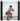# Tips in implementing Tensorflow Lite to use movenet models in C++

I built Tensorflow and Tensorflow Lite from source in order to write my own C++ implementation of a model that uses Movenet to track up to 6 skeletons. I've included some tips on how to implement and interpret the model in this documentation.

Michael Edgcumbe · 1 minute read

There are several implementations of Movenet in the wild built for Python, the web, raspberry pi, iOS, Android, etc., but finding a C++ implementation that shows how to open the model, copy an image into the input tensor, and re-interpret the results into a skeleton nodes from the output tensor requires pulling together documentation from different sources. Below are the installation steps

1. Install tensorflow from source (docs). Instead of following the last step for python, build with 'bazel build tensorflow_cc.dll' and 'bazel build tensorflow_cc.lib'
2. Install tensorflow-lite with cmake (docs)
3. Add tensorflow build products in the bazel-bin folder to the user PATH
4. Change tensorflow project to use c++ 20 standard

Once tensorflow and tensorflow-lite are installed, the next step is to set up the program to read in a bitmap image file (encoded from photoshop in 24 bit depth using BGR uint8_t channels). The image file needs to be decoded into RGB int32_t input tensor. Image height and image width need to be a multiple of 32 and no bigger than 256 on the largest size, so I resized the input image in photoshop to a bitmap of 256 x 256.

After loading the model using the tensorflow-lite interpreter (docs), we need to resize the input tensor before allocating the tensors on the model.

``````interpreter->ResizeInputTensor(input, { 1, image_width, image_height, 3 });
``````

Then, get a mutable pointer to the input tensor's typed data and copy the decoded bitmap RGB array into the typed input tensor.

``````uint8_t* typedInputTensor = interpreter->typed_input_tensor<uint8_t>(input);
for (int ii = 0; ii < in.size(); ii++) {
typedInputTensor[ii] = in[ii];
}
``````

The output tensor comes in the shape of [1,6,56], and most Python implementations reshape this into a [6,17,3] tensor/array by using numpy. Since that is not available without adding a library in c++, my VS2019 solution first unravels the data of floats into flat array and then copies those floats into a vector shaped in the [people / joints / coordinate + confidence] configuration.

Below is the sample output data for a resized version of the input image into a 256 x 256 square image:Reshaped array size: (number of people) 6 Person Data size: (number of joints) 17

##### nose

y coordinate: 43.1959 x coordinate: 104.527 confidence: 0.71908

##### left eye

y coordinate: 35.5635 x coordinate: 111.077 confidence: 0.663622

##### right eye

y coordinate: 37.1297 x coordinate: 102.99 confidence: 0.734936

##### left ear

y coordinate: 37.3665 x coordinate: 129.487 confidence: 0.913192

##### right ear

y coordinate: 38.1035 x coordinate: 110.545 confidence: 0.733949

##### left shoulder

y coordinate: 67.8285 x coordinate: 148.238 confidence: 0.760208

##### right shoulder

y coordinate: 70.6572 x coordinate: 113.235 confidence: 0.782177

##### left elbow

y coordinate: 103.372 x coordinate: 116.976 confidence: 0.771361

##### right elbow

y coordinate: 99.093 x coordinate: 75.52 confidence: 0.599049

##### left wrist

y coordinate: 68.1098 x coordinate: 91.1617 confidence: 0.6747

##### right wrist

y coordinate: 69.5191 x coordinate: 81.5573 confidence: 0.488354

##### left hip

y coordinate: 131.448 x coordinate: 186.783 confidence: 0.808038

##### right hip

y coordinate: 132.244 x coordinate: 149.793 confidence: 0.74378

##### left knee

y coordinate: 152.912 x coordinate: 150.159 confidence: 0.603105

##### right knee

y coordinate: 144.769 x coordinate: 105.615 confidence: 0.863145

##### left ankle

y coordinate: 224.04 x coordinate: 170.388 confidence: 0.88596

##### right ankle

y coordinate: 201.919 x coordinate: 121.049 confidence: 0.406041

Finished running inference